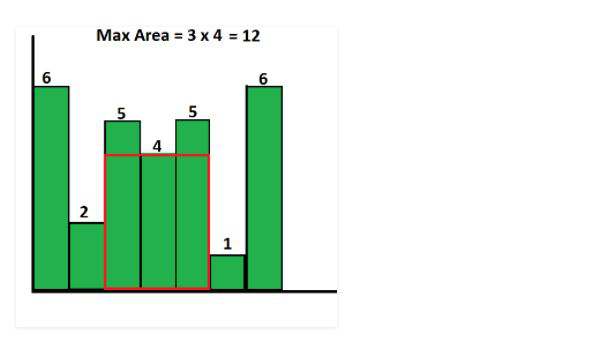Related Articles

# Largest Rectangle Area under Histogram using JavaScript | Without using Stacks

• Last Updated : 22 Sep, 2021

Find the largest rectangular area possible in a given histogram where the largest rectangle can be made of a number of contiguous bars. For simplicity, assume that all bars have the same width and the width is 1 unit.

For example, consider the following histogram with 7 bars of heights {6, 2, 5, 4, 5, 1, 6}. The largest possible rectangle possible is 12 (see the below figure, the max area rectangle is highlighted in red)Approach:

• For every bar ‘x’, we calculate the area with ‘x’ as the smallest bar in the rectangle. We will find the index of the first smaller (smaller than ‘x’) bar on left of ‘x’ and the index of first smaller bar on right of ‘x’. Let us call these indexes as ‘left index’ and ‘right index’ respectively.
• Multiply ‘x’ with “right_index-left_index-1” and store it in area
• Return max area

Below is the implementation of the above approach.

## index.js

 ``

Output:

`12`

Complexity: We are using linear search to find the minimum value, then the worst-case time complexity of this algorithm becomes O(n^2).

Hey geek! The constant emerging technologies in the world of web development always keeps the excitement for this subject through the roof. But before you tackle the big projects, we suggest you start by learning the basics. Kickstart your web development journey by learning JS concepts with our JavaScript Course. Now at it’s lowest price ever!

My Personal Notes arrow_drop_up